: The Linear Josephus Problem : The Self-Similarity of the Josephus Problems. : The Josephus Problem in Both Directions.

# Linear Josephus Problem.

The next one is the linear Josephus Problem.

Definition 3.1   Letand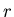be natural numbers such that. We putnumbers on a line. We start counting up from the 1st number and move from left to right removing everyth number. We change the direction when we reach the end of the line. Then we begin removing everyth number again. We denote bythe position of the last one that remains.

This problem had been studied for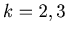in . The authors have also studied this problem from a different point of view, and they have discovered many interesting graphs that have self-similarity.

Example 3.1   This is a Mathematica program to calculate. The following Mathematica functionreturns the value of.
```jL[n_, rs_] :=
Block[{p, u}, elim = {};
p = Join[Range[n], Sort[Table[m, {m, 2, n - 1}], Greater]];
Do[Do[u1 = First[p];
u2 = First[RotateLeft[p, 1]];
If[u1 == u2,
p = RotateLeft[p, 2], p = RotateLeft[p, 1]], {s,1,rs - 1}];
u = First[p];
p = Rest[p]; elim = Append[elim, u];
If[MemberQ[p, u],
p = Drop[p, Position[p, u][]],], {t, 1, n - 1}];
p[]];
```

By using the Mathematica function in Example 3.1 we can make the graph of the list {JL(n,k), n = 1,2,3,... }.

Example 3.2   Graph 3.1 and Graph 3.2 are the graphs of the lists {, n = 1,2,..,1024} and {, n = 1,2,..,256}. Please compare these two graphs．They have the same shape, and hence these graphs seem to have Fractal behavior (self-similarity). Note that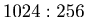=.

Graph 3.1Graph 3.2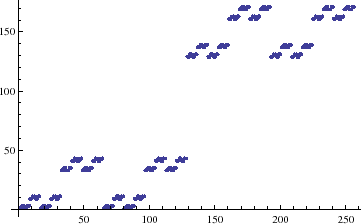Example 3.3   Graph 3.3 and Graph 3.4 are the graphs of the lists {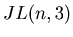, n = 1,2,..,1024} and {, n = 1,2,..,455}. Please compare these two graphs．They have the same shape, and hence these graphs have Fractal behavior (self-similarity). Note thatis approximately equal to.

Graph 3.3Graph 3.4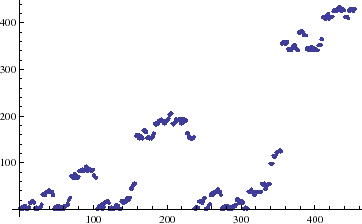: The Linear Josephus Problem : The Self-Similarity of the Josephus Problems. : The Josephus Problem in Both Directions.